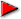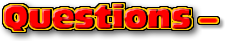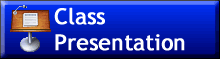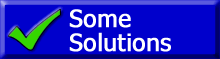ChaptersIntroductionThis text is meant to accompany class discussions. It is not everything there is to know about the basics of torque and the two conditions of equilibrium. It is meant as a prep for class. More detailed notes and examples are given in the class notes, presentations, and demonstrations (click here.)Click for the questions that go with this reading
 Page Topic 1 Definition of "capacitance" 1 Parallel plate capacitor 1 Dielectric and dielectric breakdown voltage 2 Stored energy 3 Schematics and identifying series and parallel 4 Capacitors in parallel ...calculations 4 Capacitors in series ...calculations 5 Reducing a compound circuit of capacitors to an equivalent capacitor. 5 Find the potential difference across and the charge on each capacotor in a compound circuit 5 Calculating the energy stored in a circuit of only capacitors. 6 Applications of the rules for parallel and series circuits.01: Capacitance and capacitor basics with some simple applications02: The beginning of single power source circuits using capacitors. Describes how to determine parallel and series circuits and the charge and voltage across each capacitor.03: Conceptual applications of paralell and series capacitors parts of circuits.